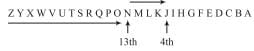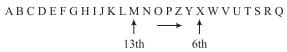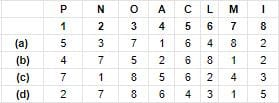# Test: Alphabet Test- 1

## 10 Questions MCQ Test Cyber Olympiad for Class 9 | Test: Alphabet Test- 1

Description
Attempt Test: Alphabet Test- 1 | 10 questions in 20 minutes | Mock test for Class 9 preparation | Free important questions MCQ to study Cyber Olympiad for Class 9 for Class 9 Exam | Download free PDF with solutions
QUESTION: 1

### If the English alphabet is written in reverse order then what will be the 4th letter to the right of 13th letter from the left?

Solution:

As per question∴ J is required latter.

QUESTION: 2

### If 1st and 26th, 2nd and 25th, 3rd and 24th and so on, letters of English alphabet are paired then when of the following pair is correct?

Solution:

According to the question AZ, BY, CX, DW, EV, FU, GT, HS, IR, JQ, KP, LO, MN.

QUESTION: 3

### In the given arrangement which letter is 10th to the right of the letter which is exactly the middle letter between F & D? F J M P O W R N B E Y C K A V L D G X U H Q I S Z T

Solution:
QUESTION: 4

If the last 10 letters of English alphabet and written in reverse order. Which of the following will be 6th to the right of 13th from the left end?

Solution:QUESTION: 5

Select the combination of numbers so that the letters arranged accordingly will form a meaning word.Solution:

C O M P L A I N
5 3 7 1 6 4 8 2

QUESTION: 6

Unscramble the letters in the words given and find the odd one out.

Solution:

EARTH, VENUS, MOON, MARS.
All are planets.

QUESTION: 7

If T = 20; NET = 39 then NUT = ?

Solution:

T = 20
NET = 14 + 5 + 20 = 39
NUT = 14 + 21 + 20 = 55

QUESTION: 8

In each of the following questions find out how many such pairs of letters are there in the given word each of which has as many letters between them in the word as in English alphabet?

BRIGHTER

Solution:
QUESTION: 9

In each of the following questions find out how many such pairs of letters are there in the given word each of which has as many letters between them in the word as in English alphabet?

PRODUCTION

Solution:
QUESTION: 10

In each of the following questions find out how many such pairs of letters are there in the given word each of which has as many letters between them in the word as in English alphabet?

CORPORATE

Solution:Use Code STAYHOME200 and get INR 200 additional OFF Use Coupon Code